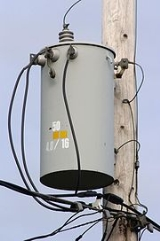xTransformerOverview

A transformer is a device that transfers electrical energy from one circuit
Electrical network
An electrical network is an interconnection of electrical elements such as resistors, inductors, capacitors, transmission lines, voltage sources, current sources and switches. An electrical circuit is a special type of network, one that has a closed loop giving a return path for the current...

to another through inductively coupled
Inductive coupling
In electrical engineering, two conductors are referred to as mutual-inductively coupled or magnetically coupled when they are configured such that change in current flow through one wire induces a voltage across the ends of the other wire through electromagnetic induction...

conductors—the transformer's coils. A varying current
Electric current
Electric current is a flow of electric charge through a medium.This charge is typically carried by moving electrons in a conductor such as wire...

in the first or primary winding creates a varying magnetic flux
Magnetic flux
Magnetic flux , is a measure of the amount of magnetic B field passing through a given surface . The SI unit of magnetic flux is the weber...

in the transformer's core and thus a varying magnetic field
Magnetic field
A magnetic field is a mathematical description of the magnetic influence of electric currents and magnetic materials. The magnetic field at any given point is specified by both a direction and a magnitude ; as such it is a vector field.Technically, a magnetic field is a pseudo vector;...

through the secondary winding. This varying magnetic field induces
Electromagnetic induction
Electromagnetic induction is the production of an electric current across a conductor moving through a magnetic field. It underlies the operation of generators, transformers, induction motors, electric motors, synchronous motors, and solenoids....

a varying electromotive force (EMF)
Electromotive force
In physics, electromotive force, emf , or electromotance refers to voltage generated by a battery or by the magnetic force according to Faraday's Law, which states that a time varying magnetic field will induce an electric current.It is important to note that the electromotive "force" is not a...

, or "volt
Volt
The volt is the SI derived unit for electric potential, electric potential difference, and electromotive force. The volt is named in honor of the Italian physicist Alessandro Volta , who invented the voltaic pile, possibly the first chemical battery.- Definition :A single volt is defined as the...

age", in the secondary winding. This effect is called inductive coupling
Inductive coupling
In electrical engineering, two conductors are referred to as mutual-inductively coupled or magnetically coupled when they are configured such that change in current flow through one wire induces a voltage across the ends of the other wire through electromagnetic induction...

.

If a load is connected to the secondary, current will flow in the secondary winding and electrical energy will be transferred from the primary circuit through the transformer to the load.